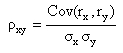# Can we code this?

in the first part i wanted to find the correlation between two different stocks

and in second part i wanted to do simple linear regression of these two stocks, to find out ‘Residual term’ and ‘Predicted Y’. so i can use this to forecast the price.

Is it possible to code this in Pi?

and also can we backtest econometrics models (like AR, MA, ARMA, etc) in Pi (like R or MATLAB).

Thanks,

Mahesh

The correlation coefficient is calculated as:you can do it in excel, it can not be coded in tradescript

yes, but exporting data to excel is not possible everytime when i’m considering large number of stocks, like if i want find correlation between Nify 50 stocks to Nifty indices.